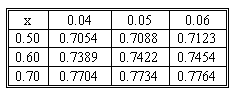### CFA Practice Question

There are 227 practice questions for this study session.

### CFA Practice Question

A stock sells for \$110. A put option on the stock has an exercise price of \$105 and expires in 43 days. If the continuously compounding interest rate is 0.11 and the standard deviation of the stock's returns is 0.25, what is the price of the put option according to the Black-Scholes-Merton model?

Below is the relevant part of the cumulative probabilities table for a standard normal distribution.A. 1.46
B. 2.32
C. 2.78
Explanation: d1 = {ln(110/105) + [0.11 + (0.252/2)] x (43/365)} / (0.25 (43/365)1/2) = 0.7361
d2 = 0.7361 - 0.25 x (43/365)1/2 = 0.6503
N(d1) = N(0.74) = 0.7704
N(d2) = N(0.65) = 0.7422
p = \$105 x e -0.11 x (43/365) x (1 - 0.7422) - \$110 x (1 - 0.7704) = \$1.46